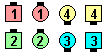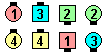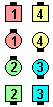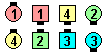Definitions of Square Dance Calls and Concepts
Change Lanes [C3B]

Index -->  Plus  |  A1  |  A2  |  C1  |  C2  |  C3A  |  C3B  |  C4  |  NOL  |
Definitions (Text Only) -->  Plus  |  A1  |  A2  |  C1  |  C2  |  C3A  |  C3B  |  C4  |  NOL  |
 Find call:

 \$B8@8l(BCenters \$B\$,(B Mini Wave \$B\$N(B Box \$B\$H\$J\$C\$F\$\$\$kJ?9T\$J(B line \$B\$^\$?\$OB>\$NE,@Z\$J(B formation \$B\$+\$i(B.

Centers \$B\$O(B Any Hand Remake & Spread \$B\$r\$7(B, Ends \$B\$O(B Circulate & Crossover Circulate \$B\$r\$7\$^\$9(B.

\$BJ?9T\$J(B Line \$B\$+\$i\$OJ?9T\$J(B Line \$B\$G=*\$o\$j\$^\$9(B.

Parallel Two-Faced Lines \$B\$+\$i(B:Change Lanes\$B\$NA0(B \$B8e(B
3/4 Box \$B\$+\$i(B:Change Lanes\$B\$NA0(B \$B8e(B

Retain Your Lane [obsolete,C4] : Centers \$B\$O(B Any Hand Remake \$B\$r\$7(B, Ends Circulate twice \$B\$r\$7\$^\$9(B. Retain Your Lane \$B\$O(B Change Lanes & Spread \$B\$H%8%g!<%/\$G\$\$\$o\$l\$^\$9(B.

fraction Chain & Circulate In [C4]: \$BE,@Z\$J(B formation \$B\$+\$i(B. Centers \$B\$O(B Cast Off \$B\$rM?\$(\$i\$l\$?(B fraction \$B\$@\$19T\$\$(B; Very Centers Trade; Centers Cast Off 3/4 & Spread \$B\$r\$7(B, Ends \$B\$O(B Circulate and Crossover Circulate \$B\$r\$7\$^\$9(B. Change Lanes \$B\$O(B 1/4 Chain & Circulate In \$B\$HF1\$8\$G\$9(B.Choreography for Change LanesComments? Questions? Suggestions?

https://www.ceder.net/def/changelanes.php?level=master&language=japan
27-January-2020 13:13:29